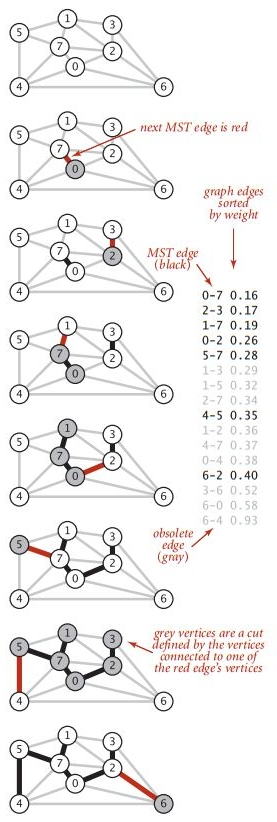# 《算法》笔记 11 - 最小生成树

• 最小生成树的应用
• 切分定理
• 贪心算法
• 加权无向图的数据结构
• Prim算法
• Kruskal算法

### 切分定理

#### 切分定理

• 用一条边连接树中的任意两个顶点都会产生一个新的环；
• 从树中删去任意条边都将会得到两颗独立的树。
接下来用反证法证明切分定理：令e为权值最小的横切边，T为图的最小生成树，假设T不包含e，那么将e加入T得到的图必然含有一条经过e的环，且这个环至少还有另一条横切边——设为f，f的的权重必然大于e（因为e的权重是最小的且图中所有边的权值都不相同）。那么删去f保留e就可以得到一颗权值更小的树，这与假设矛盾。

### 加权无向图的数据结构

#### 带权重的边Edge的实现

public class Edge implements Comparable<Edge> {
private final int v;
private final int w;
private final double weight;

public Edge(int v, int w, double weight) {
this.v = v;
this.w = w;
this.weight = weight;
}

public double weight() {
return this.weight;
}

public int either() {
return this.v;
}

public int other(int vertex) {
if (v == vertex)
return w;
if (w == vertex)
return v;
else
throw new RuntimeException("Inconsistent edge");
}

public int compareTo(Edge that) {
if (this.weight() < that.weight())
return -1;
else if (this.weight() > that.weight())
return 1;
else
return 0;
}

public String toString() {
return String.format("%d-%d %.2f", v, w, weight);
}
}


either和other方法可以返回边连接的两个端点，weight表示边的权重。

#### 加权无向图EdgeWeightedGraph的实现

public class EdgeWeightedGraph {
private static final String NEWLINE = System.getProperty("line.separator");
private final int V; // vertex
private int E; // edge

public EdgeWeightedGraph(int V) {
this.V = V;
this.E = 0;
for (int v = 0; v < V; v++) {
}
}

public EdgeWeightedGraph(In in) {
for (int i = 0; i < E; i++) {
Edge e = new Edge(v, w, weight);
}
}

public int V() {
return V;
}

public int E() {
return E;
}

int v = e.either(), w = e.other(v);
E++;
}

}

public String toString() {
StringBuilder s = new StringBuilder();
s.append(V + " vertices, " + E + " edges " + NEWLINE);
for (int v = 0; v < V; v++) {
s.append(v + ": ");
for (Edge w : adj[v]) {
s.append(w + " | ");
}
s.append(NEWLINE);
}
return s.toString();
}

public Bag<Edge> edges() {
Bag<Edge> b = new Bag<Edge>();
for (int v = 0; v < V; v++) {
for (Edge w : adj[v]) {
}
}
return b;
}


### Prim算法

8
16
4 5 0.35
4 7 0.37
5 7 0.28
0 7 0.16
1 5 0.32
0 4 0.38
2 3 0.17
1 7 0.19
0 2 0.26
1 2 0.36
1 3 0.29
2 7 0.34
6 2 0.40
3 6 0.52
6 0 0.58
6 4 0.93• 将顶点0添加到最小生成树之中，将它的邻接链表中的所有边添加到优先队列之中。
• 将顶点7和边0-7添加到最小生成树之中，将顶点的邻接链表中的所有边添加到优先队列之中。
• 将顶点1和边1-7添加到最小生成树之中，将顶点的邻接链表中的所有边添加到优先队列之中。
• 将顶点2和边0-2添加到最小生成树之中，将边2-3和6-2添加到优先队列之中。边2-7和1-2失效。
• 将顶点3和边2-3添加到最小生成树之中，将边3-6添加到优先队列之中。边1-3失效。
• 将顶点5和边5-7添加到最小生成树之中，将边4-5添加到优先队列之中。边1-5失效。
• 从优先队列中删除失效的边1-3、1-5和2-7。
• 将顶点4和边4-5添加到最小生成树之中，将边6-4添加到优先队列之中。边4-7和0-4失效。
• 从优先队列中删除失效的边1-2、4-7和0-4。
• 将顶点6和边6-2添加到最小生成树之中，和顶点6相关联的其他边均失效。

public class LazyPrimMST {
private boolean[] marked;
private Queue<Edge> mst;
private MinPQ<Edge> pq;

public LazyPrimMST(EdgeWeightedGraph G) {
pq = new MinPQ<Edge>();
marked = new boolean[G.V()];
mst = new Queue<Edge>();
visit(G, 0);
while (!pq.isEmpty()) {
Edge e = pq.delMin();
int v = e.either(), w = e.other(v);
if (marked[v] && marked[w])
continue;

mst.enqueue(e);
if (!marked[v])
visit(G, v);
if (!marked[w])
visit(G, w);
}
}

public void visit(EdgeWeightedGraph G, int v) {
marked[v] = true;
for (Edge e : G.adj(v)) {
if (!marked[e.other(v)]) {
pq.insert(e);
}
}
}

public Iterable<Edge> edges() {
return mst;
}

// cmd /c --% java algs4.four.LazyPrimMST ..\..\..\algs4-data\tinyEWG.txt
public static void main(String[] args) {
In in = new In(args);
EdgeWeightedGraph ewg = new EdgeWeightedGraph(in);
LazyPrimMST lazyPrim = new LazyPrimMST(ewg);
double weight=0;
for (Edge e : lazyPrim.edges()) {
weight += e.weight();
StdOut.println(e);
}
StdOut.println(weight);
}
}


visit()方法的作用是为树添加一个顶点，将它标记为“已访问”，并将与它关联的所有未失效的边加入优先队列中。在while循环中，会从优先队列取出一条边，如果它没有失效，就把它添加到树中，否则只是将其从优先队列删除。然后再根据添加到树中的边的顶点，更新优先队列中横切边的集合。

### Kruskal算法

Prim算法是一条边一条边地来构造最小生成树，每一步都为一棵树添加一条边。接下来要学习的Kruskal算法处理问题的方式则是按照边的权重顺序，从小到大将边添加到最小生成树中，加入的边不会与已经加入的边构成环，直到树中含有V-1条边为止。从一片由V颗单结点的树构成的森林开始，不断将两棵树合并，直到只剩下一颗树，它就是最小生成树。

【】

public class KruskalMST {
private Queue<Edge> mst;
private double _weight = 0;

public KruskalMST(EdgeWeightedGraph G) {
mst = new Queue<Edge>();
MinPQ<Edge> pq = new MinPQ<Edge>();
UF uf = new UF(G.V());

for (Edge e : G.edges()) {
pq.insert(e);
}

while (!pq.isEmpty() && mst.size() < G.V() - 1) {
Edge e = pq.delMin();
int v = e.either(), w = e.other(v);
if (uf.connected(v, w))
continue;
uf.union(v, w);
mst.enqueue(e);
_weight += e.weight();
}
}

public Iterable<Edge> edges() {
return mst;
}

public double weight() {
return _weight;
}

// cmd /c --% java algs4.four.KruskalMST ..\..\..\algs4-data\tinyEWG.txt
public static void main(String[] args) {
In in = new In(args);
EdgeWeightedGraph ewg = new EdgeWeightedGraph(in);
KruskalMST kruskalMST = new KruskalMST(ewg);
for (Edge e : kruskalMST.edges()) {
StdOut.println(e);
}
StdOut.println(kruskalMST.weight());
}
}


posted @ 2020-01-01 16:43  zhixin9001  阅读(...)  评论(...编辑  收藏